Geometry Fundamentals

What is $x?$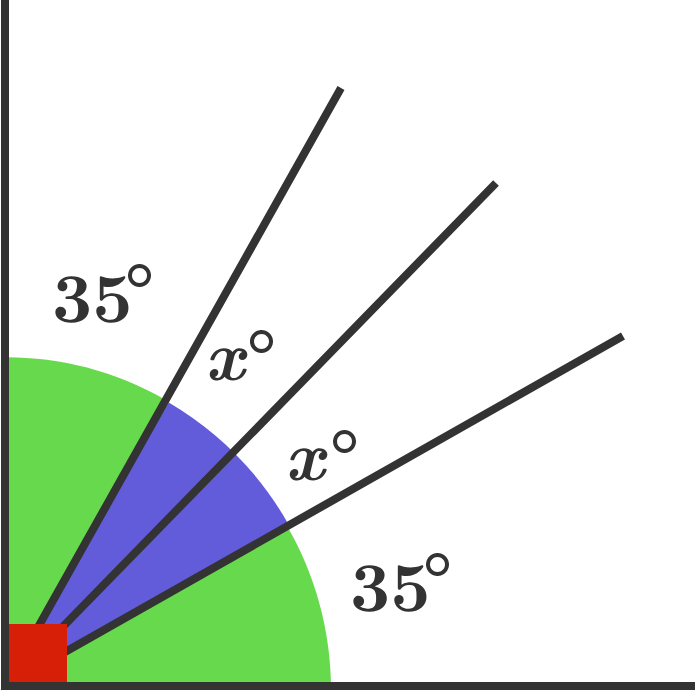Angle Hunt

What is the missing angle?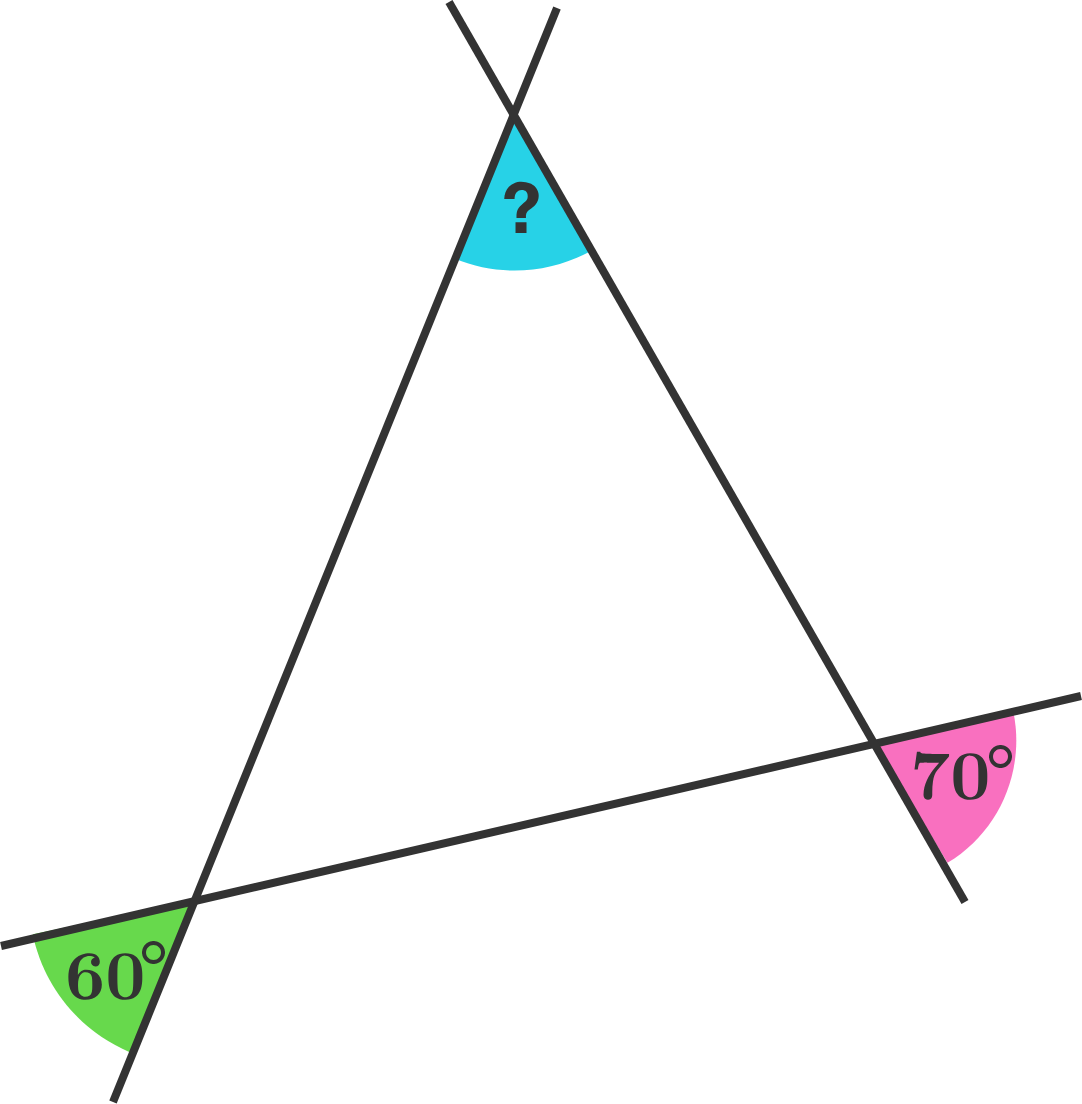Angle Hunt

What is the measure of the green angle?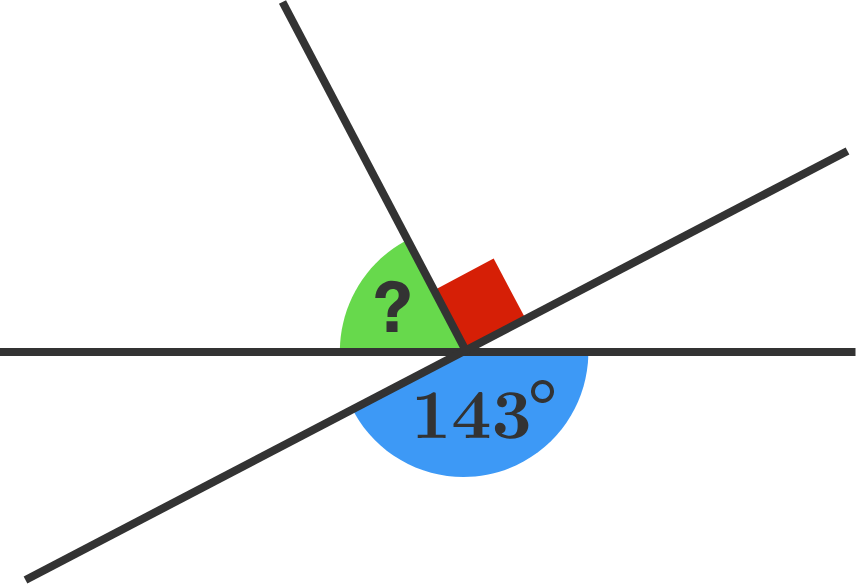Angle Hunt

Seven unknown angles are each marked with a question mark. How many of the angles with question marks measure $55^\circ?$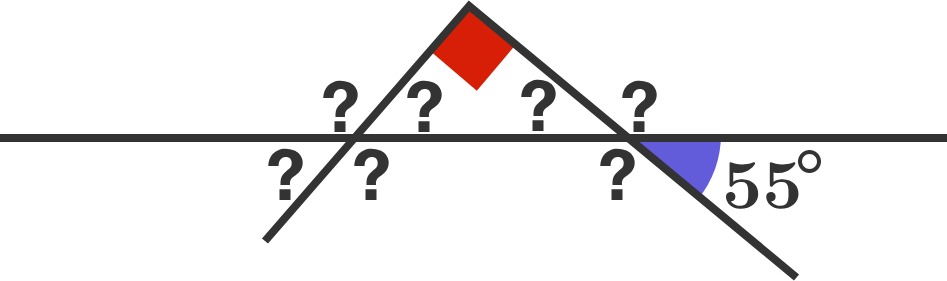Angle Hunt

Three squares are added to the sides of a triangle, as shown.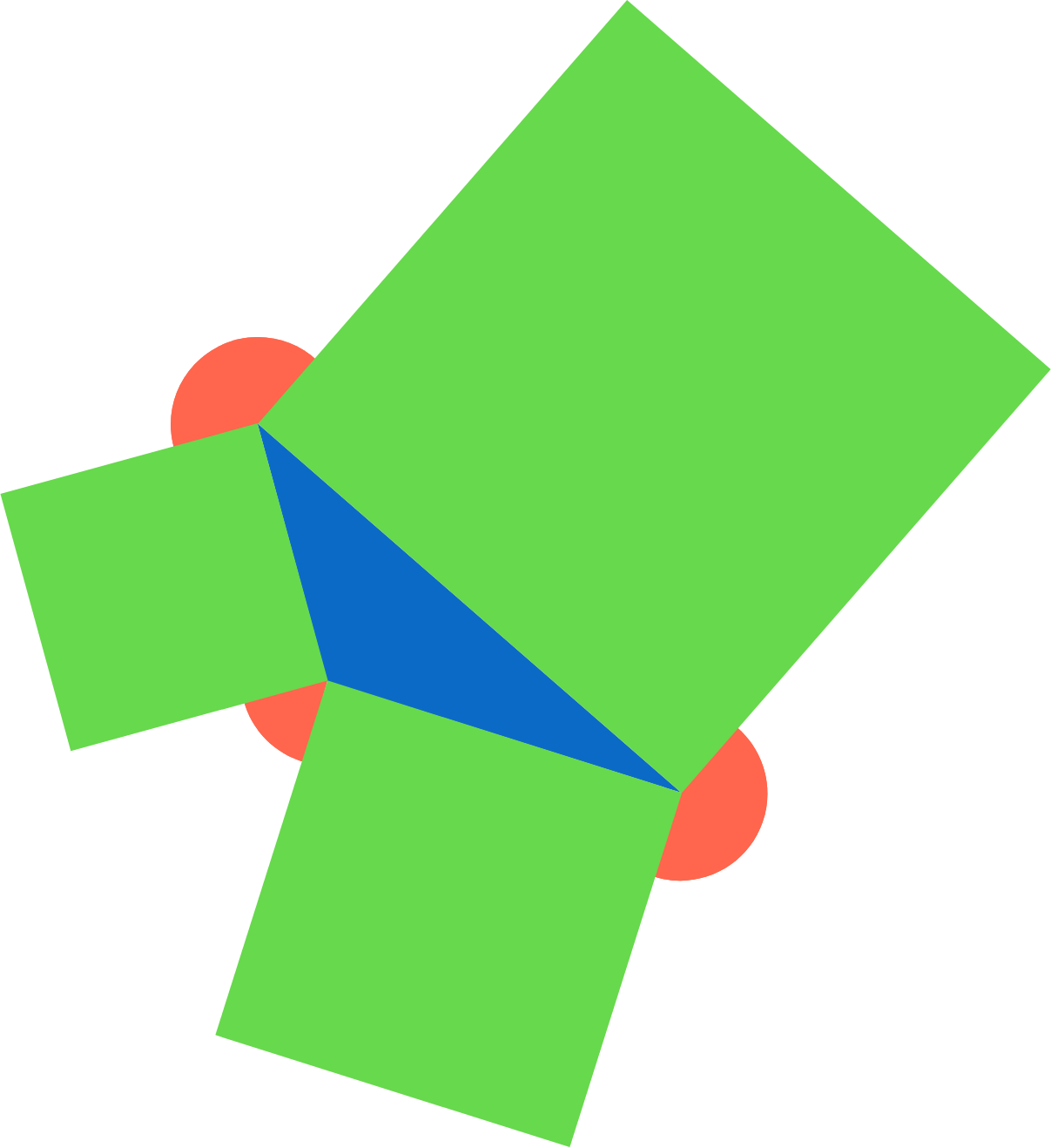Is the following statement true or false?

The sum of the three red angles will always equal $360^\circ$ regardless of the type of triangle drawn.

×